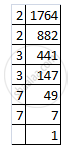Share

# Find the Square Root the Following by Prime Factorization. 1764 - CBSE Class 8 - Mathematics

ConceptSquare Roots - Finding Square Root Through Prime Factorisation

#### Question

Find the square root  the following by prime factorization.

1764

#### Solution

Resolving 1764 into prime factors:
1764 = 2 x 2 x 3 x 3 x 7 x 7Grouping the factors into pairs of equal factors:
1764 = (2 x 2) x (3 x 3) x (7 x 7)
Taking one factor for each pair, we get the square root of 1764:
2 x 3 x 7 = 42

Is there an error in this question or solution?

#### APPEARS IN

Solution Find the Square Root the Following by Prime Factorization. 1764 Concept: Square Roots - Finding Square Root Through Prime Factorisation.
S